Math resources Algebra

Math equations

# Math equations

Here you will learn about math equations, including what they are and how to solve them.

Students will first learn about math equations as part of expressions and equations in 6th grade. They continue to expand upon this knowledge in 7th and 8th grade.

## What are math equations?

Math equations are statements made up of algebraic expressions and an equal sign. Math equations include defined math formulas that can be solved with substitution.

• Math formulas are rules that connect two or more variables. They are used to work out the values of unknown variables by substituting in other known values.

For example, here is a rectangle with base b and height h.

The area of any rectangle can be found with the formula b \times h.

Step-by-step guide: Math formulas

• Substitution is when you give variables in an algebraic expression, equation or inequality a value. You use substitution to identify solutions.

For example,

Does x = 2 make the equation 5x+2=14 true?

Substitute 2 for x and follow the order of operations:

5 \times 2+2=14

10+2=14

12 14

Substituting 2 for x, does not make the equation true, so 2 is not a solution of the equation 5x+2=14.

Step-by-step guide: Substitution

• Math equations also include multi step equations and equations with fractions.

One step equations are algebraic equations with a single variable that can be solved with one step.

In order to solve, you will need to isolate the variable (get it alone) on one side of the equation. This can be done with a model or by using the inverse operation.

For example, 3x =15

Using a model:Using inverse operations:

Sort the (+1) tiles so an equal amount
are with each (x) tile.

There are five (+1) tiles for each (x) tile.
x=5

3x=15

Get the variable alone by using the
inverse operation. The inverse
operation of multiplication is division.
Divide 3 on both sides of the equation.

\cfrac{3x}{3}=\cfrac{15}{3}

Since \cfrac{3}{3} \,=1, the left side can be written
as 1x or x.

The right side of the equation can be
written as \cfrac{15}{3}=5.

x=5

substituting the value for x into the
original equation.

\begin{aligned} 3x&=15\\ 3(5)&=15 \; \color{#77dd77} ✔ \end{aligned}

Step-by-step guide: One step equations

• Solving equations with fractions is when the unknown is part of the numerator and/or denominator of a fraction.

To solve equations with fractions, apply the inverse operation to both sides of the equation – a strategy also referred to as the “balance method.”

The inverse operation of division is multiplication.

For example, \, \cfrac{x+3}{3}=8

3\left(\cfrac{x+3}{3}\right)=3(8) \quad *Multiply each side by 3.

x+3=24

x = 21

Step-by-step guide: Solve equations with fractions

### What are math equations?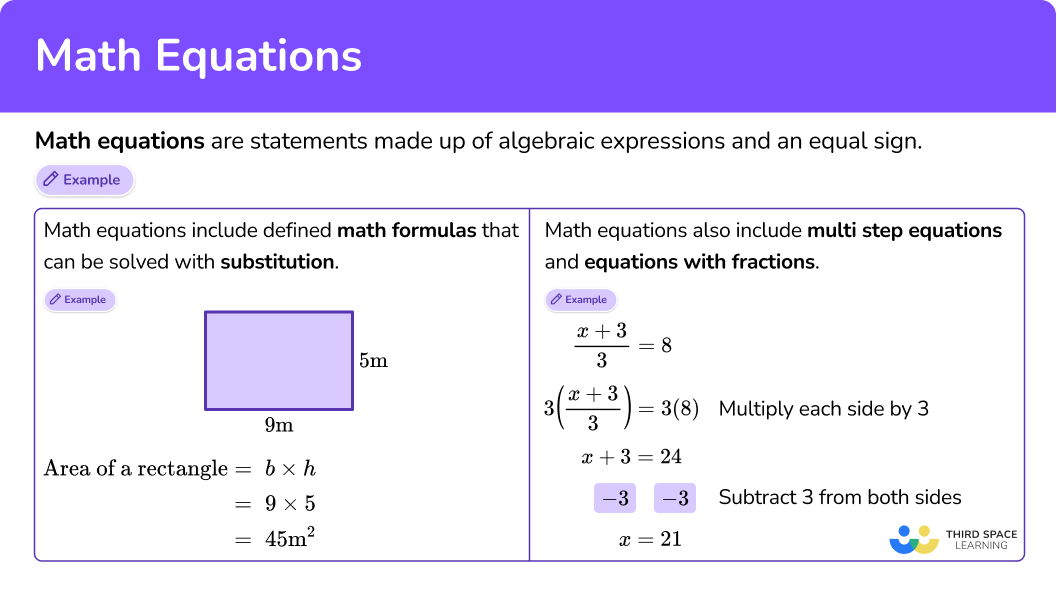## Common Core State Standards

• Grade 6 – Expressions and Equations (6.EE.A.2c)
Write, read, and evaluate expressions in which letters stand for numbers.

• Grade 6 – Expressions and Equations (6.EE.B.5)
Understand solving an equation or inequality as a process of answering a question: which values from a specified set, if any, make the equation or inequality true? Use substitution to determine whether a given number in a specified set makes an equation or inequality true.

• Grade 7 – Expressions and Equations (7.EE.A.1)
Apply properties of operations as strategies to add, subtract, factor, and expand linear expressions with rational coefficients.

• Grade 8 – Expressions and Equations (8.EE.C.7)
Solve linear equations in one variable.

• Grade 8 – Expressions and Equations (8.EE.C.7b)
Solve linear equations with rational number coefficients, including equations whose solutions require expanding expressions using the distributive property and collecting like terms.

## How to solve with math equations

There are a lot of ways to use math equations. For more specific step-by-step guides, check out the pages linked in the “What are math equations?” section above or read through the examples below.

## Math equations examples

### Example 1: volume of a cube

Find the volume of the cube using the formula, ​​V=s \times s \times s or V=s^3

1. Use the formula given in the question.

Use the formula ​​V=s \times s \times s to find the volume of the cube.

The sides of the cube are all 8. Substitute the measurements into the given formula.

V=8 \times 8 \times 8

2Work carefully to answer the question, one step at a time.

\begin{aligned} & V=8 \times 8 \times 8 \\\\ & V=64 \times 8 \\\\ & V=512 \end{aligned}

The final answer is 512 units^3.

### Example 2: substitution basic inequality

Does r=-2 make the inequality r \leq-11 true?

-2 \leq-11

There is nothing to calculate, but you read the inequality ‘-2 is less than or equal to -11.’ Also consider the inequality on a number line.

Since -2 is not less than or equal to -11, substituting -2 for r makes the inequality false. This means -2 is NOT a solution for r \leq-11.

*Note that there are an infinite amount of solutions for this inequality – any number less than or equal to -11 makes the inequality true.

### Example 3: substitution equation

Find the value of e \, (114-t) when e=11 and t=58.

11 \, (114-58)

11 \, (114-58)

=11 \, (56) \quad \quad **Remember 11 \, (56) is the same as 11 \times 56.

=616

### Example 4: solve for the variable using addition/subtraction

Solve the equation for x.

8+x=-13

8+x=-13

Because of the commutative property, this equation could also be written as:

x+8=-13 → inverse operation is subtraction because subtraction undoes addition.

8+x =-13

On the left side of the equation 8-8=0, leaving just 0+ x, which is the same as x.

On the right side of the equation:

\begin{aligned} x= & -13 \\\\ & -8 \\\\ x= & -21 \end{aligned}

### Example 5: solve the equation using multiplication/division

Solve the equation, \, \cfrac{x}{5}=-20 for x.

\cfrac{x}{5}=-20 → inverse operation of division is multiplication.

\cfrac{x}{5} \times 5=-20 \times 5

On the left side of the equation: \cfrac{x}{5} \times 5=\cfrac{5 x}{5}=1 x

On the right side of the equation: -20 \times 5=-100

\begin{aligned} \cfrac{x}{5} \times 5 & =-20 \times 5 \\\\ 1 x & =-100 \\\\ x & =-100 \end{aligned}

### Example 6: solving equations with fractions – two steps

Solve for x\text{: } \cfrac{x-9}{8}=6.5

The unknown is x.

Looking at the left hand side of the equation, 9 is subtracted from x and then divided by 8 (the denominator of the fraction).

\cfrac{x-9}{8}

First, multiplying both sides of the equation by 8.

Then, add 9 to each side.

8\left(\cfrac{x-9}{8}\right)=(6.5) \, 8 \quad *Multiply each side by 8.

x-9=52

x = 61

You can check the answer by substituting the answer back into the original equation.

\cfrac{x-9}{8}=\cfrac{61-9}{8}=52 \div 8=6.5

### Teaching tips for math equations

• Instead of introducing this topic through worksheets and rote memorization of the rules, use manipulatives, such as algebra tiles. This can help students build conceptual understanding of these abstract concepts.

However, it is important not to rush the work with these tools – while it may take more time in the short term to develop understanding with manipulatives versus memorizing a procedure, in the long term students will be more flexible and more easily able to adapt their strategies to more complex topics.

**Note: It is also useful to discuss the limitations of algebra tiles with students, such as they can only be used to represent smaller integers.

• Whenever possible, consider using project based learning or real-life assessments, such as having students measure items within the classroom and then using the formulas to calculate the area of the items.

• When teaching students how to solve math problems such as these, the traditional focus is often solely on the solution of an equation. While this is a crucial piece, also give students time to explore what numbers are not a part of the solution set as well.

In middle school, students are still building foundational ideas around algebraic expressions, equations and inequalities, so it is important not to always rush them to the answer.

• Always have students check to make sure their answer is correct by substituting the value back into the original equation. This helps them catch simple mistakes and gives them practice substituting values into the equation.

### Easy mistakes to make

• Thinking the variable can only be on the left-hand side
While the variable is commonly seen on the left side of the equation, it can also be on the right-hand side.

For example,
\begin{aligned} & 19=3 x \\ & \cfrac{19}{3}=\cfrac{3}{3} x \\ & 6 \cfrac{1}{3}=x \end{aligned}

• Confusing the difference between a mathematical expression and a mathematical equation
A mathematical expression is a statement that does not include an equal sign, like 43-x. A mathematical equation is a complete mathematical sentence that includes an equals sign, like 43-x=22.

• Forgetting to follow the order of operations (PEMDAS)
When substituting values into an equation to solve, always follow the order of operations: parentheses first, then exponents, then from left to right multiplication and division, and then from left to right addition and subtraction.

For example,
Solve 5x-10 \div 2 when x=2.

• Forgetting that the solution to an equation can be any type of number
The unknowns do not have to be whole numbers or integers. In fact, the solutions often involve fractions or decimals.

### Practice math equations questions

1. Find the area of the triangle using the appropriate formula.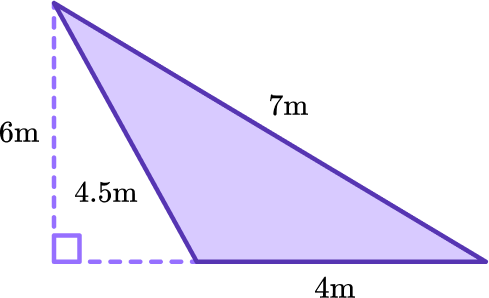24 \, m^218 \, m^212 \, m^263 \, m^2Use the formula A=\cfrac{1}{2} \, b h to find the area of the triangle.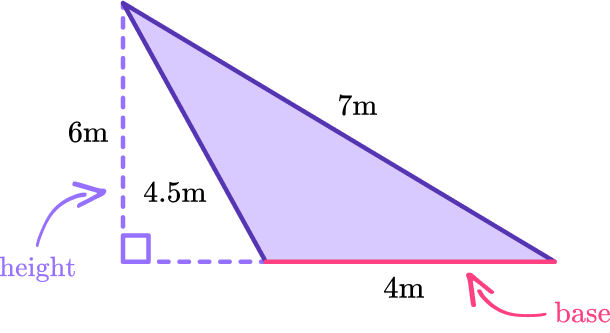Substitute the measurements into the formula and solve.

\begin{aligned} & A=\cfrac{1}{2} \, (4 \times 6) \\\\ & A=\cfrac{1}{2} \, (24) \\\\ & A=12 \end{aligned}

The area of the triangle is 12 \, m^2.

2. Find the value of 45+88x when x=13.

1,7298,8588,8131,189Substitute each variable with its given value.

45+88 \times 13

Solve using the order of operations.

\begin{aligned} & 45+88 \times 13 \\\\ & =45+1,144 \\\\ & =1,189 \end{aligned}

3. Which number is a solution for t > -56 \, ?

-55-56-57-258Substitute each variable with its given value and then read each inequality:

-55 > -56… ‘-55 is greater than -56’

-56 > -56… ‘-56 is greater than -56’

-57 > -56… ‘-57 is greater than -56’

-258 > -56… ‘-258 is greater than -56’

Substituting -55 for t makes the inequality true, so -55 is a solution for t > -56.

4. Solve the equation for x \text{: } -4x=22

x= 22x= -5.5x= -5x= 4.5-4x=22 → The inverse operation is division, so divide both sides of the equation by -4.

\cfrac{-4 x}{-4}=\cfrac{22}{-4}

On the left side of the equation: \cfrac{-4 x}{-4}=1 x and 1 x=x

On the right side of the equation: \cfrac{22}{-4}=-5.5

\begin{aligned} & \cfrac{-4 x}{-4}=\cfrac{22}{-4} \\\\ & x=-5.5 \end{aligned}

Check:5. Solve the equation for p \text{: } p-\cfrac{4}{5}=\cfrac{1}{3}

x=\cfrac{17}{30}x=\cfrac{3}{2}x=\cfrac{15}{17}x=1 \, \cfrac{2}{15}p-\cfrac{4}{5}=\cfrac{1}{3} → The inverse operation is addition, so add \, \cfrac{4}{5} \, to both sides of the equation.

p-\cfrac{4}{5}+\cfrac{4}{5}=\cfrac{1}{3}+\cfrac{4}{5}

On the left side of the equation: -\cfrac{4}{5}+\cfrac{4}{5}=0, so p-0 is left.

On the right side of the equation: \cfrac{1}{3}+\cfrac{4}{5}=\cfrac{5}{15}+\cfrac{12}{15}=\cfrac{17}{15}

\begin{aligned} & p-\frac{4}{5}+\frac{4}{5}=\frac{1}{3}+\frac{4}{5} \\\\ & p-0=\frac{17}{15} \\\\ & p=\frac{17}{15} \text { or } 1 \frac{2}{15} \end{aligned}

Check: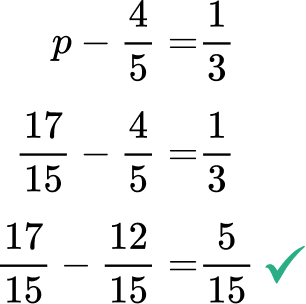6. Solve: \cfrac{6 x-9}{3}=1

x=-\cfrac{1}{2}x=-2x=\cfrac{1}{2}x=2First, multiply both sides of the equation by 3.

Next, add 9 to both sides.

Finally, divide both sides by 6.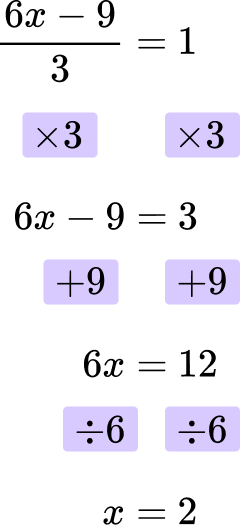The final answer is x = 2.

You can check the answer by substituting the answer back into the original equation.

\cfrac{6 \, \times \, 2-9}{3}=\cfrac{12-9}{3}=\cfrac{3}{3}=1

## Math equations FAQs

Why are math formulas important?

Math formulas make work in mathematics more efficient.

While students always spend ample time working to build ideas around a mathematical concept, formalizing it with the use of a formula (like the area of a triangle as A=\cfrac{1}{2} \, b h) allows them to move forward in their understanding and answer more complex questions.

Growing shape patterns start with a shape and grow in a constant way from term to term. Students learn how to write general rules for these patterns. For example, Start with 2 circles and add 3 circles each time.” This can then be translated into a math equation for the pattern: 2 + 3n = t , where n = the pattern term number and t = the total number of circles.

When do students learn the Pythagorean theorem?

What types of equations do students learn about in mathematics?

Students start with simple equations and math formulas in elementary school and then progress to solving linear equations and systems of equations in middle school.

In high school, students continue to expand their knowledge by learning more advanced equations such as quadratic equations (including the quadratic formula), exponential equations, and trigonometric equations.

As they move into college level courses such as precalculus and calculus, they will solve rational equations, logarithm equations, parametric equations and differential equations

## Still stuck?

At Third Space Learning, we specialize in helping teachers and school leaders to provide personalized math support for more of their students through high-quality, online one-on-one math tutoring delivered by subject experts.

Each week, our tutors support thousands of students who are at risk of not meeting their grade-level expectations, and help accelerate their progress and boost their confidence.